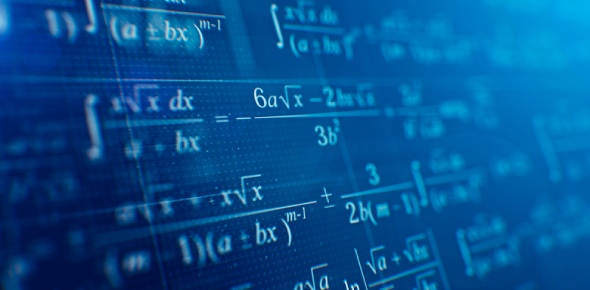# Trivia Quiz On Mathematics #7

5 Questions | Total Attempts: 5560SettingsMathematics may not interest you if you’re not actively seeking a job which requires it, but it’s never a bad thing to brush up on a little mathematical knowledge every now and again. Whether you need to study for an upcoming math exam, or you simply want to challenge yourself and potentially boost your knowledge on a certain subject, this trivia quiz on mathematics should do the trick!

Related Topics
• 1.
What is the number pi?
• A.

3,14

• B.

2,79

• 2.
In whic lection is cosine
• 3.
What is E=mc2
• A.

Formula of nuclear bomb

• B.

Physic formula of gravity

• C.

Weight formula

• 4.
Is it true thet is a2=b1
• A.

Yes

• B.

No

• 5.
Which formula is for extent square